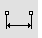# DistanceAnalyze

Distance

The Distance command reports the distance between two points.

#### Steps

1. Pick the first point.
2. Pick the second point.
The distance between the points displays in the command history window, with angles and distances in the axis directions in world and construction plane coordinates.
Command-line options

Units

Set the units for the distance display.

##### Nesting the Length, Radius, and Distance commands

The Length, Radius, and Distance commands are nestable. This means that if Rhino is asking for a distance in a command, you can use these commands to measure another object or distance and the resulting value will be feed to the command as if you had typed the number.

#### Example using Offset

1. Start the OffsetSrf command.
2. At the prompt for the offset distance, type Length, Radius, or Distance, and press Enter.
3. Select a curve.
The offset distance will be set to that value.

#### Example using Fillet

1. Start the Fillet command.
2. At the prompt for the radius, type Length, Radius, or Distance, and press Enter.
3. Select a curve.
The fillet radius will be set to that value.